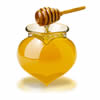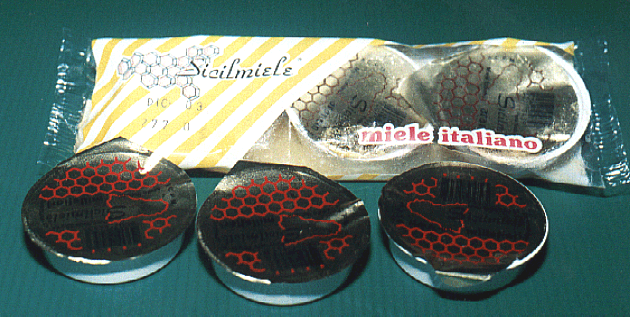### B=document.all; C=document.layers; T1=new Array("images/ape_animata2.gif",58,55,"images/ape_animata2.gif",30,31,"images/ape_animata2.gif",28,26,"images/ape_animata2.gif",22,21,"images/ape_animata2.gif",16,16,"images/ape_animata2.gif",10,10) nos=parseInt(T1.length/3) rate=50 ie5fix1=0; ie5fix2=0; for (i=0;i<nos;i++){ createContainer("CUR"+i,i*10,i*10,i*3+1,i*3+2,"","<img src='"+T1[i*3]+"' width="+T1[(i*3+1)]+" height="+T1[(i*3+2)]+" border=0>")} function createContainer(N,Xp,Yp,W,H,At,HT,Op,St){ with (document){ write((!B) ? "<layer id='"+N+"' left="+Xp+" top="+Yp+" width="+W+" height="+H : "<div id='"+N+"'"+" style='position:absolute;left:"+Xp+"; top:"+Yp+"; width:"+W+"; height:"+H+"; "); if(St){ if (C) write(" style='"); write(St+";' ") } else write((B)?"'":""); write((At)? At+">" : ">"); write((HT) ? HT : ""); if (!Op) closeContainer(N) } } function closeContainer(){ document.write((B)?"</div>":"</layer>") } function getXpos(N){ return (B) ? parseInt(B[N].style.left) : C[N].left } function getYpos(N){ return (B) ? parseInt(B[N].style.top) : C[N].top } function moveContainer(N,DX,DY){ c=(B) ? B[N].style :C[N];c.left=DX;c.top=DY } function cycle(){ //if (IE5) if (document.all&&window.print){ ie5fix1=document.body.scrollLeft; ie5fix2=document.body.scrollTop; } for (i=0;i<(nos-1);i++){ moveContainer("CUR"+i,getXpos("CUR"+(i+1)),getYpos("CUR"+(i+1))) } } function newPos(e){ moveContainer("CUR"+(nos-1),(B)?event.clientX+ie5fix1:e.pageX+2,(B)?event.clientY+ie5fix2:e.pageY+2 ) } if(document.layers) document.captureEvents(Event.MOUSEMOVE) document.onmousemove=newPos setInterval("cycle()",rate) var ErrMsg = "tasto destro disattivato "; function disableRightClick(btnClick) { if (navigator.appName == "Netscape" && btnClick.which == 3) // check for netscape and right click { alert(ErrMsg); return false; } else if (navigator.appName =="Microsoft Internet Explorer" && event.button == 2) // for IE and Right Click { alert(ErrMsg); return false; } } document.onmousedown = disableRightClick;The monodoses
 Monodoses are 25 g trays. To be used by hotels, communities etc .. In addition, a tris box for supermarkets was created.